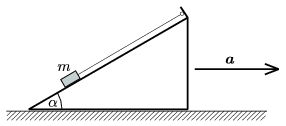Mathematical and Physical Journal
for High Schools
Issued by the MATFUND Foundation
 Already signed up? New to KöMaL?

#Problem P. 4034. (January 2008)

P. 4034. A body of mass m=1.8 kg is placed to an inclined plane, the angle of inclination is=30o, and is attached to the top end of the slope wit a thread which is parallel to the slop. Then the slope is moved with a horizontal acceleration of a. Friction is negligible.

a) Find the acceleration if the body pushes the slope with a force of.

b) Find the tension.(4 pont)

Deadline expired on February 11, 2008.

### Statistics:

 205 students sent a solution. 4 points: 150 students. 3 points: 20 students. 2 points: 8 students. 1 point: 8 students. 0 point: 15 students. Unfair, not evaluated: 4 solutionss.

Problems in Physics of KöMaL, January 2008